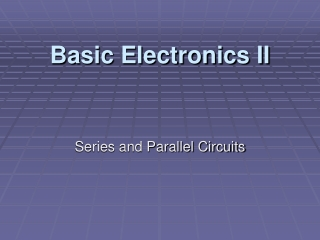DownloadDownload PresentationBasic Electronics II

# Basic Electronics II

Download Presentation## Basic Electronics II

- - - - - - - - - - - - - - - - - - - - - - - - - - - E N D - - - - - - - - - - - - - - - - - - - - - - - - - - -
##### Presentation Transcript

1. Basic Electronics II Series and Parallel Circuits

2. Series Circuits • When components are connected in successive order. • Only one path for electron flow. • Current is the same for all series components.

3. Series Circuits

4. Total R = sum of all series resistances: • RT = R1+ R2+ R3 ...+ etc. • Where RT is the total resistance and R1, R2, R3 are individual series resistances.

5. I = ET / RT • RT is the sum of all resistances. • ET is the voltage applied across the total resistance. • I is the current in all parts of the string.

6. Series IR Voltage Drops • The IR voltage across each resistance is known as an IR drop or a voltage drop. • It reduces the potential difference available for the remaining resistance in a series circuit. • V1, V2etc are used for the voltage drops across each resistor to distinguish them from the applied voltage source ET. V1 = IT X R1, V2 = IT X R2, etc • ET = V1 + V2 + .... + etc

7. Voltage Divider • An arrangement of 2 resistors in series is often called a voltage divider. • Each IR drop V = its proportional part of the applied voltage or: • V = R / RT x ET • A potentiometer (volume control) is a voltage divider where the point of division is made variable.

8. Total Power in Series Circuits • The total power is the sum of the power dissipated in each part of the circuit or: • PT= P1 + P2 + ...+ etc • Remember: 3 Power Formulas • P = E x I • P = I2 x R • P = E2 / R

9. Effect of an open is a series circuit • Because the current is the same in each part of a series circuit - • An open results in no current for the entire circuit.

10. Parallel Circuits • Each parallel path is a branch with its own individual current. • Parallel circuits have one common voltage across all branches, however - • Individual branch currents can be different.

11. Parallel Circuits R1 = 2Ω R2 = 4Ω

12. Voltage is equal across parallel branches • Since components are directly connected across the voltage source, they must have the same potential as the source. • Therefore, the voltage is the same across components connected in parallel. • Components requiring the same voltage would be connected in parallel.

13. Each branch I = E / R • I1 = E / R1 • I2 = E / R2 and so on. • If individual resistances are the same, then individual branch currents would also be the same.

14. Main-line IT = sum of branch currents • IT= I1 + I2 + ...+ etc

15. Resistances in parallel • Total resistance across the main line can be found by Ohm’s Law: Divide the common voltage by the total current. • RT = E / IT • RT is always less than the smallest individual branch resistance

16. Reciprocal resistance formulae • 1 / RT= 1/R1+ 1/R2 + 1/R3+ ... etc • This formulae works for any number of parallel resistances of any value

17. If the values of R are the same • If all resistors in parallel are the same value, then use this shortcut: • The value of one resistor/total number of resistors = Total resistance

18. If the there are only 2 resistors of differing values • If there are only two resistors in parallel and they are different in value, then use this shortcut: • R1 x R2/R1 + R2 = Total resistance

19. Finding an unknown R • In able to find what value Rx must be added in parallel with a known R to get a required Rt • Rx RT/R - RT = Rx

20. Power in parallel circuits • Total power equals the sum of the individual power in each branch. • PT= P1 + P2 + ...+ etc • In both series and parallel circuits the sum of the individual values of power dissipated in the circuit = the total power generated by the source.

21. Parallel Current Dividers • Individual branch currents can be found without knowing the applied voltage. • Currents divide inversely as the branch resistances. • I1 =R2/R1 + R2 (IT) • I2 =R1/R1 + R2 (IT)

22. Effect of an open in a parallel circuit • An open in the main line results in no current in all branches • An open in a branch results in no current for that individual branch - other branches are not affected

23. Effect of a short circuit in parallel • A short circuit has practically zero resistance • A short results in excessive current